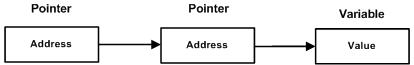#### Pointer Pointers In C Programming#### Simple Pointer Definition

Pointers in C language is a variable that stores/points the address of another variable is called pointer in C,

#### Pointer To Pointer

When one pointer variable is used to store the address of another pointer variable, therefore, such type of pointer is known as pointer to pointer variable.

Pointer to pointer variable is also called as double pointer in C programming.#### Declaration of pointer-to-pointer

```  int **ptr ;
```

Note:

• To store the address of normal variable we use single pointer variable.
• To store the address of single pointer variable we use double pointer variable.

#### Output:

``` Address of normal variable 'a' = 2293316
Address of pointer variable '*ptr1' = 2293304
Address of pointer-to-pointer '**ptr2' = 2293296
```

#### Output:

``` Address of number variable is 22fe44
Address of p variable is 22fe44
Value of *p variable is 20
Address of p2 variable is 22fe38
Value of **p2 variable is 20
--------------------------------
```